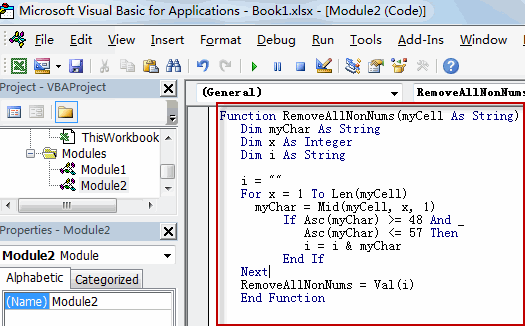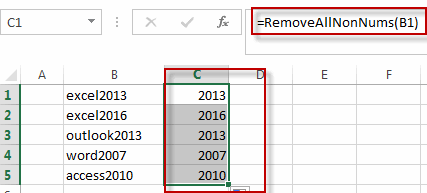# Removing Non Numeric Characters

This post will guide you how to remove non numeric characters from cells with a formula in Excel. How do I remove non-numeric characters in cells in Excel. How to remove non-numeric characters from cells with VBA Code in Excel. Or how to strip out non-numeric characters from a given cell in Excel.

## Removing Non Numeric Characters with a Formula

Assuming that you have a list of data in range B1:B5, and you want to remove all non-numeric characters from the range of cells B1:B5. How to achieve it. You can use an Excel Array formula based on the TEXTJOIN function, the IFERROR function, the ROW function and the INDIRECT function. Like this:

`=TEXTJOIN("",TRUE,IFERROR(MID(B1,ROW(INDIRECT("1:100")),1)+0,""))`

You need to Type this formula into a blank cell and then press CTRL +Shift + Enter keys to change the formula as an Array formula. and then drag the AutoFill Handle from C1 to C5.

## Removing Non Numeric Characters with User Defined Function

You can also try to write an user defined function with VBA code to remove all non numeric characters from a cell in Excel. Just do the following steps:

#1 open your excel workbook and then click on “Visual Basic” command under DEVELOPER Tab, or just press “ALT+F11” shortcut.#2 then the “Visual Basic Editor” window will appear.

#3 click “Insert” ->”Module” to create a new module.#4 paste the below VBA code into the code window. Then clicking “Save” button.```Function RemoveAllNonNums(myCell As String)
Dim myChar As String
Dim x As Integer
Dim i As String

i = ""
For x = 1 To Len(myCell)
myChar = Mid(myCell, x, 1)
If Asc(myChar) >= 48 And _
Asc(myChar) <= 57 Then
i = i & myChar
End If
Next
RemoveAllNonNums = Val(i)```

#5 back to the current worksheet, try to enter the below formula in Cell C1.

`=RemoveallNonNums(B1)`You can also use the following VBA macro to achieve the same result.

```Sub RemoveAllNonNums()
Dim myR As Range
Dim myRange As Range
Set myRange = Application.Selection
Set myRange = Application.InputBox("select one range that you want to remove non-numeric characters", RemoveAllNonNums, myRange.Address, Type:=8)
For Each myR In myRange
myOut = ""
For i = 1 To Len(myR.Value)
tmp = Mid(myR.Value, i, 1)
If tmp Like "[0-9]" Then
myStr = tmp
Else
myStr = ""
End If
myOut = myOut & myStr
Next i
myR.Value = myOut
Next
End Sub```

## Removing Numeric characters only

If you only want to remove all numeric characters from a cell in Excel, and you can try to use the following array formula based on the TEXTJOIN function, the IF function, the ISERR function, the MID function, the ROW function and the INDIRECt function.

`=TEXTJOIN("",TRUE,IF(ISERR(MID(B1,ROW(INDIRECT("1:99")),1)+0),MID(B5,ROW(INDIRECT("1:99")),1),""))`

Type this formula in a blank cell and then press Ctrl + Shift + Enter shortcuts in your keyboard, and then drag the AutoFill Handle over other cells to apply this array formula.

### Related Functions

• Excel ROW function
The Excel ROW function returns the row number of a cell reference.The ROW function is a build-in function in Microsoft Excel and it is categorized as a Lookup and Reference Function.The syntax of the ROW function is as below:= ROW ([reference])….
• Excel IF function
The Excel IF function perform a logical test to return one value if the condition is TRUE and return another value if the condition is FALSE. The IF function is a build-in function in Microsoft Excel and it is categorized as a Logical Function.The syntax of the IF function is as below:= IF (condition, [true_value], [false_value])….
• Excel MID function
The Excel MID function returns a substring from a text string at the position that you specify.The syntax of the MID function is as below:= MID (text, start_num, num_chars)…
• Excel INDIRECT function
The Excel INDIRECT function returns the cell reference based on a text string, such as: type the text string “A2” in B1 cell, it just a text string, so you can use INDIRECT function to convert text string as cell reference….

Related Posts
VBA Macro For VLOOKUP From Another Sheet

In the previous post, you should know that how to fix or remove the #N/A error when using VLOOKUP formula to lookup value from another sheet. And this post will show you how to use VBA code to vlookup data ...

How To Insert Comments in Protected Worksheet in Excel

This post will show you how to allow comments in a protected worksheet in Excel. You can easily to insert comments into cells in a normal worksheet in Excel, but if want to insert a comment in a worksheet that ...

How To Convert Text to Upper Cases(Using VBA) in Excel

This post will show you how to switch from lower case to upper case in Excel. and I am going to show you two different ways of converting text to upper cases using formula or VBA macro in Excel 2013,Excel ...

How To Hide Every Other Row in Excel (Using VBA)

This post will show you how to hide alternate rows or columns in Excel or how to hide every third, fourth, fifth row or column in Excel. If you want to hide every other row in your current worksheet, how ...

How to Disable the Save As Prompt in Excel

This post will show you how to use a VBA Macro to save an Excel file and overwrite any existing file without a prompt so that you are going to get the little window that says file already exists do ...

How to Count Cells that Contain even or odd numbers in Excel

This post will guide you how to count the number of cells that contain odd or even numbers within a range of cells using a formula in Excel 2013/2016.How do I count cells that contain odd numbers through the use ...

How to Count Cells that Contain negative Numbers in Excel

This post will guide you how to count the number of cells that contain negative numbers within a range of cells using a formula in Excel 2013/2016.You can count the number of negative numbers in your data using easy functions ...

How to Count Cells Are Not Blank or Empty in Excel

This post will guide you how to count cells that are not blank or empty in a given range cells using a formula in Excel 2013/2016.How do I count the number of cells that are not blank in a particular ...

How to Count Cells Less Than a Specific Value in Excel

This post will guide you how to count the number of cells less than a particular numeric value in a given range cells using a formula in Excel 2013/2016. How do I count cells that are less than a specific ...

How to Count Cells Greater Than a Specific Value in Excel

This post will guide you how to count the number of cells greater than a particular numeric value in a given range cells using a formula in Excel 2013/2016. How do I count cells that are greater than a specific ...

Sidebar